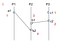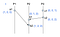# Let’s study distributed systems — 2. ClockFrom: Flickr

Did you read my previous post — Let’s study distributed systems 1. Introduction? I tried to tell you some introduction to distributed systems.

In this article, I want to talk about clock. In our daily life, many things are based on time. When we meet our friends, when a train starts… but it assumes that we are sharing the same clock.

# Clocks are not accurate

Most clocks in this world are not accurate. Even if it is a radio clock, it doesn’t guarantee that the clock is perfectly accurate. Radio clock uses radio time signal internally, but its speed is the same as light. It is estimated that if the place which radio time signal emitted is 186 miles away, there will be 1 millisecond late.

However, in our real world, 1 ms late doesn’t matter. Even if a teacher says that tomorrow’s class will be started from 09:00, it might be 09:01 or 09:00:30. Our clock is not accurate, but we have few chances which introduces us problem caused by it.

# Clocks in computers

To get accurate time as much as possible, there is NTP (Network time protocol). Each computer fetches time from a NTP server and set it as the computer’s time. However, the same problem like network latency might cause inaccurate clock even if we are using NTP.

# Why does accurate time matter?

Suppose, we are developing a ticket ordering system. A client will send a request to server to order a ticket. This time, the server received 2 requests in very short time. Let’s say reqA and reqB. The server need to know which request is sent earlier because there is only 1 remaining ticket. The server records the time when a request comes in. In this case, that recorded time might not tell us which request is earlier. Because of network latency, request order might be changed during internet trip. In other words, even if reqA arrived to the server earlier than reqB, it doesn’t guarantee that reqA is sent earlier than reqB.

If we have accurate clocks in both client and server, client can send timestamp with ticket ordering request. However, because computers cannot have accurate clock, there is no way to know which request is earlier exactly.

Like above example, we sometimes need to know the event’s happened-before relations, especially in distributed systems. If above example happened in just one process, inaccurate clock won’t be a problem.

# Heppened-before relations

Heppened-before relations is a relation between two events, which indicates that which event happened before the other event. It necessarily doesn’t mention that one event was really executed before the other event. In other words, the real world’s execution event can be ignored because handling real world execution order is not easy. What is important is that if event order is consistent, it’s OK. When event a happens before event b, it’s written as a b.

There are 3 basic theory about happened-before; transitivity, irreflexivity and antisymmetry. (Note: “a ↛ b” means that a is not before b)

• if ab and b → c then a → c

If a is before b and b is before c then a is before c. (transitivity)

• a ↛ a

No event happen before itself. (irreflexivity)

• a → b then b ↛ a

If a is before b, b is not before a (antisymmetry)

In logical clock, this can happen;

• a ↛ b and b ↛ a

a is not before b and b is not before a.

In this case, event a and event b run in parallel. Parallel doesn’t mean that these 2 events are run actually in parallel. It just mentions that nobody can guarantee which event is earlier, and regarding that they are run in parallel doesn’t have any contradictions.

For example, suppose event a happened on process A, and event b on process B. In this case, if process A and B never connected, we cannot assume which event is before the other one.

# Logical Clock

We can define events’ order by using heppend-before relation. However, using happend-before to define events’ order is not efficient because it requires us to save information about transitivity. To work more efficiently, there is a virtual clock which is based on heppend-before idea, called logical clock.

In logical clock algorithm, set t(e) as the virtual time of an event e. t represents the time, but it’s not the one in our real world but just a sequential number. It can define order of events. It works like this;

• Initially, t = 0 in each process
• When an event e which is not a message receiving event happens, increment t. Then, set t as t(e)
• When a message sending event e happens, attach t(e) to the message.
• When a message receiving event e happens, set max(current time, attached time to e) as t(e)

Using these rules, it can guarantee that if e → e’ then t(e) < t(e’).How logical clock works

Let’s see above image as an example. Because of if a → b and b → c then a → c, we can know each happend-before relation. Red character represents t(e).

# A problem of logical clock, and Vector clock

The known problem of logical clock is that it just guarantees if e → e’ then t(e) < t(e’), but it doesn’t guarantee that if t(e) < t(e’) then e → e’ . Let’s see above image again. t(a1) is less then t(c2). However, it does not guarantee that a1 → c2.

Vector clock can resolve the problem; in other words, it can guarantee both of above.

In vector clock, each process has an array of time. Length of the array is the number of processes. Suppose number of processes is n, i ∈ {1, 2, …, n} then a process Pi has a Vector clock Vi = (v1, v2, … vn).

• Initially, t = 0 in each process. Therefore, each vector clock V = (0, 0, …, 0).
• When an event e which is not message receiving event happens, process Pi defines V’ from current vector clock V = (v1, v2, …, vn) by changing it as vi ← vi+1. Then, V’ will be the time V(e) which e happened
• When an event e which is message sending event happens, attach V(e) to the message
• When an event e which is message receiving event happens, set vj ← max(vj, vj’) (1 ≤ j ≤ n) and vi ← vi + 1 to attached time then set it as V(e)How vector clock works

This is how vector clock works in above example. In vector clock, when there are 2 vector clock V = (v1, v2, … vn) and V’ = (v’1, v’2, …, v’n), if arbitary i which is i ∈ {1, 2, …, n} can be vi ≤ v’i and at least one i can be vi < v’i then V < V’.

# Conclusion and What’s next?

Thank you for reading through my article! In this article, we learned why clock matters in distributed systems, and some clock theories. This looks very basic content, but it’s actually very important concept. When you create your own distributed database, you will need to think about clock.

Next, I’m planning to write something about distributed snapshot. If you like this article please clap, follow me, and share to others!

Hidetatsu loves infrastructures, database, concurrent programming, transactions, distributed systems… https://github.com/dty1er https://dtyler.io

Hidetatsu loves infrastructures, database, concurrent programming, transactions, distributed systems… https://github.com/dty1er https://dtyler.io